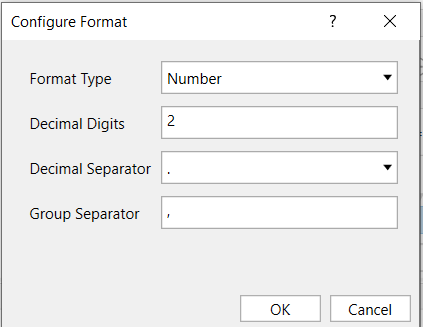# How to enforce two digits after decimal point on a decimal variable

Hello, I have a decimal variable that I need to have two digits after the decimal point. Until now the process is giving me:

8.00 = 8
1.25 = 1.25
1.20 = 1.2
10000.00 = 10000
I need all values with two digits and in the decimal format, how can I do it?

Regards,

Alamyr

double num
Convert.ToDouble(String.Format(“{0:0.00}”, num))
or
String.Format(“{0:0.00}”, 123.4567);
or

``````double source = 1234.56789;
// 1234.57
var result = source.ToString("F2");
``````
6 Likes

@Alamyr_Junior

You can use format value activity

You can set your format as belowMark as solution if this helps

Thanks

2 Likes

@Alamyr_Junior

Hope the solution works for you

Mark as solution if this helps

Thanks

this worked for me, thank you so much

1 Like

This topic was automatically closed 3 days after the last reply. New replies are no longer allowed.